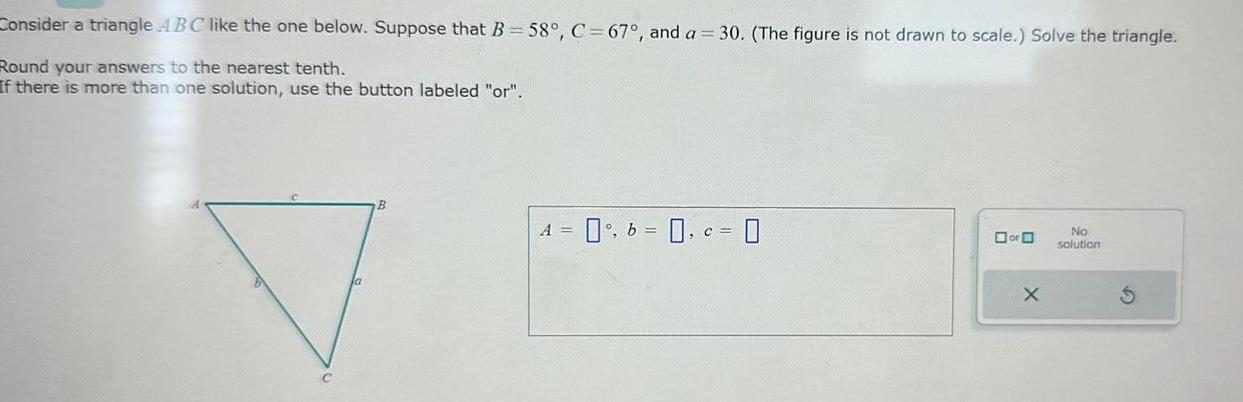Question:

# Consider a triangle ABC like the one below Suppose that B 58

Last updated: 9/19/2023Consider a triangle ABC like the one below Suppose that B 58 C 67 and a 30 The figure is not drawn to scale Solve the triangle Round your answers to the nearest tenth If there is more than one solution use the button labeled or A 0 b 0 c 0 or O X No solution